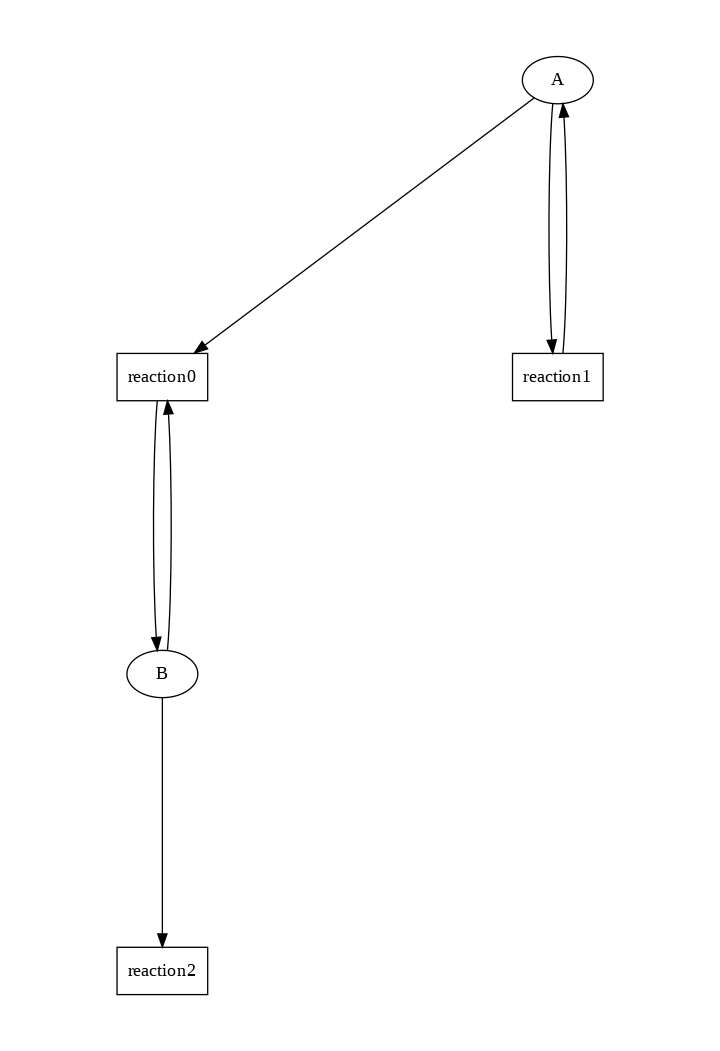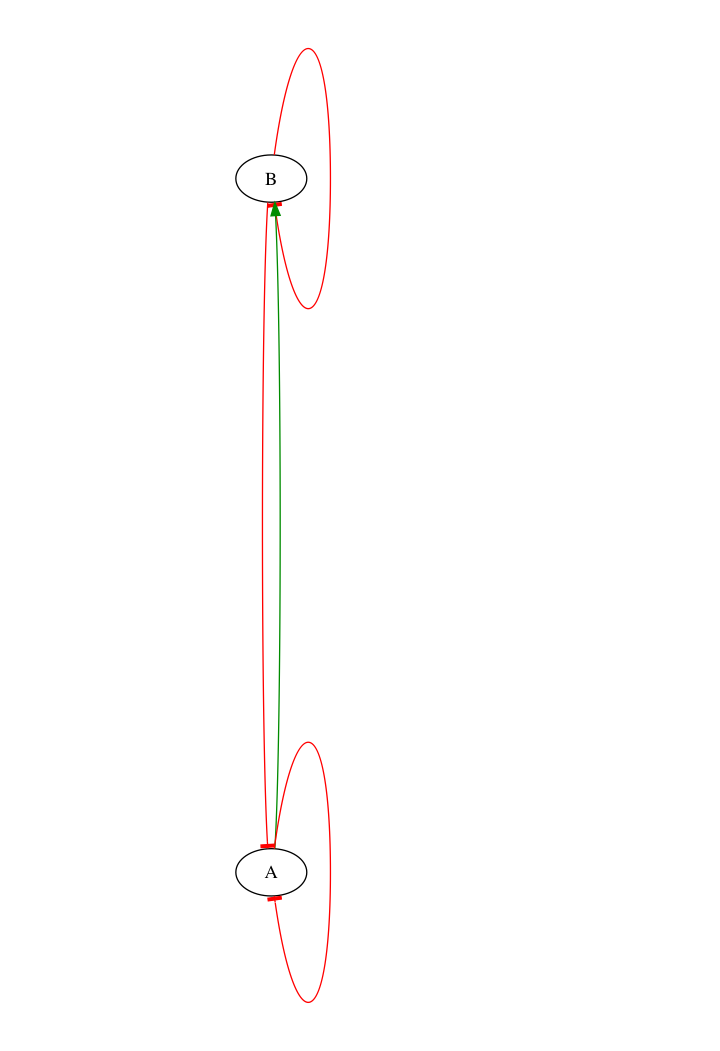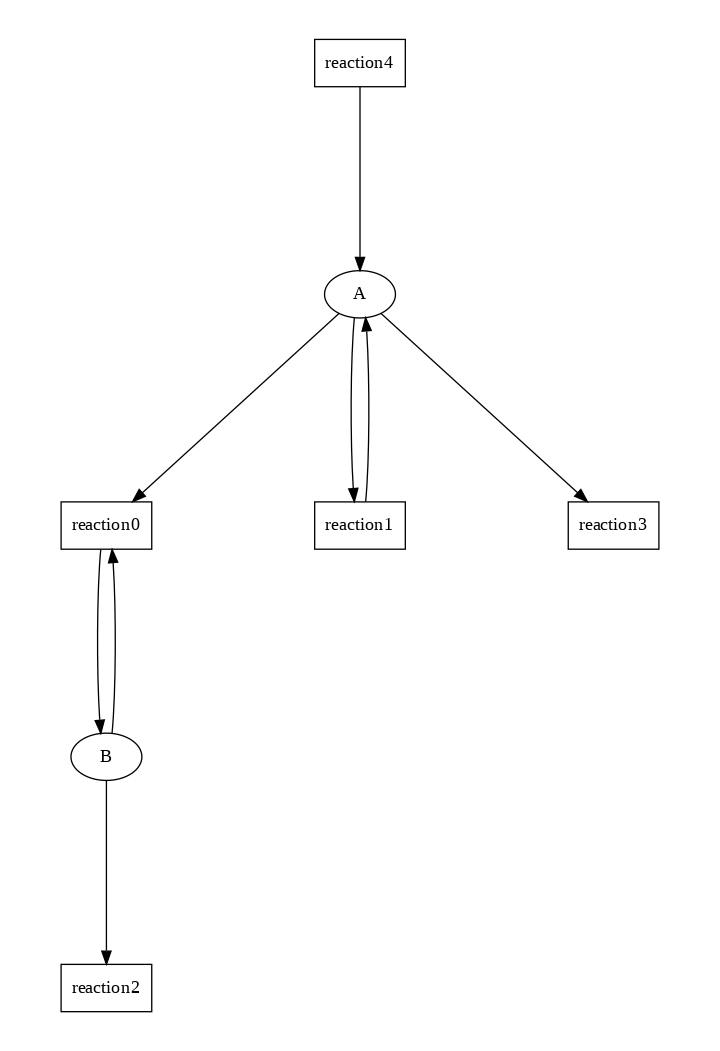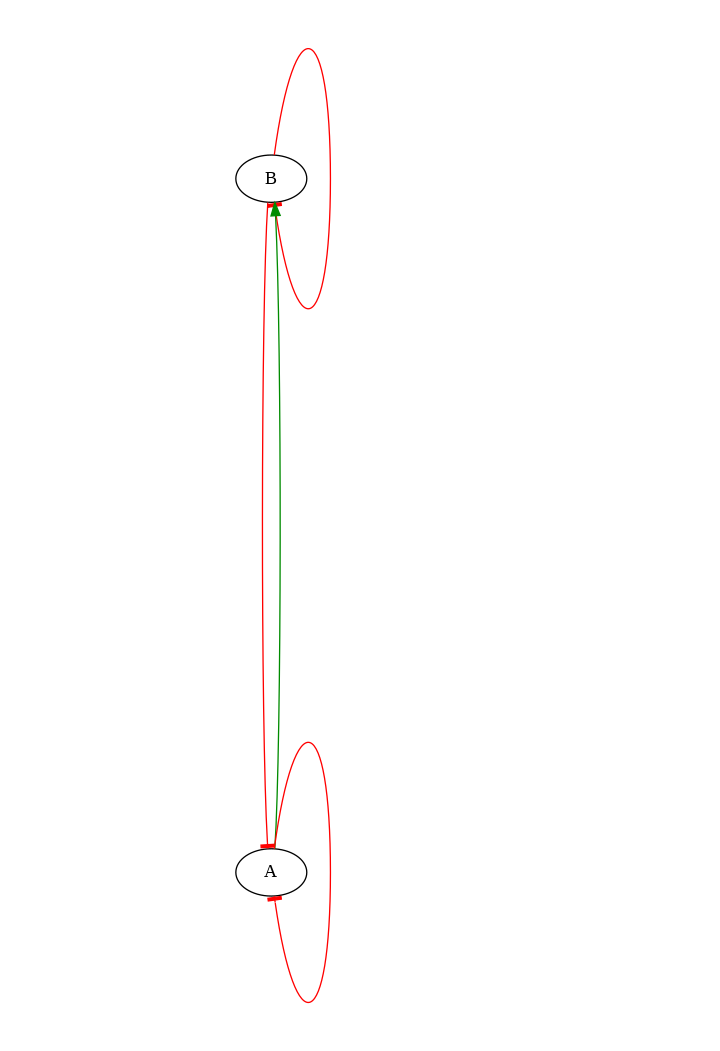# TD1: Lotka-Volterra's prey-predator enzymatic model¶

• reaction network
• differential, stochastic and boolean semantics
• addition of immigration/emigration reactions

F. Fages, 18 Jan. 2019

In :
clear_model.

In :
present(A,a). present(B,b).

In :
k1*A*B for A+B => 2*B.

In :
k2*A for A => 2*A.

In :
k3*B for B => _.

In :
parameter(a=1, b=1, k1=2, k2=2, k3=1).

In :
list_model.

Out:
k1*A*B for A=[B]=>B.
k2*A for _=[A]=>A.
k3*B for B=>_.
present(A,a).
present(B,b).
parameter(
a = 1,
b = 1,
k1 = 2,
k2 = 2,
k3 = 1
).

In :
draw_reactions.In :
draw_influences.## Continuous semantics¶

• One observes sustained oscillations and limit cycle
In :
list_ode.

\begin{align*} B_0 &= 1\\ A_0 &= 1\\ a &= 1\\ b &= 1\\ k1 &= 2\\ k2 &= 2\\ k3 &= 1\\ \frac{dB}{dt} &= k1*A*B-k3*B\\ \frac{dA}{dt} &= k2*A-k1*A*B\\ \end{align*}
In :
search_conservations.

Out:
No complex invariant found

In :
option(time:40).

In :
numerical_simulation. plot.

In :
plot(show:B, against:A).


## Stochastic semantics¶

• With this parameter set, the prey and predator extend almost surely
• The intuitive explanation is that low concentrations become almost surely 0 at some time point in the stochastic simulation.
In :
seed(0). numerical_simulation(method:ssa). plot.

In :
plot(show:B, against:A).


## Boolean semantics¶

• reason just on the presence or absence of molecular species
• asynchronous Boolean transition system ignoring reaction rates
• SAT algorithm for enumerating all stable states, here:
• either both A, B absent is a stable state
• or B absent A present
• yet both A and B present is not stable since A and B may disappear in this case
In :
list_stable_states.

Out:
[A-0,B-0]
[A-1,B-0]

• Computation Tree Logic (CTL) symbolic model-checking algorithm for enumerating possible qualitative behaviors of interest, here:
• both A and B may extinguish
• species A may survive with no possibility of disappearing (reachability of stable state A present)
• B may survive but with always the possibility of disappearing (reachability of non stable steady state B present)
• no Boolean oscillation possible (0 is stable)
• checkpoints are possibly non causal phenomenological properties
In :
generate_ctl_not.

Out:
reachable(stable('A'))
reachable(stable(not'A'))
reachable(stable(not'B'))
checkpoint2('B',not'A')
checkpoint2('A',not'B')

In :
expand_ctl(stable(f)).

Out:
AG(f)

In :
expand_ctl(steady(f)).

Out:
EG(f)

In :
expand_ctl(reachable(steady(s))).

Out:
EF(EG(s))

In :
expand_ctl(checkpoint(x,y)).

Out:
not EU(not x,y)

In :
expand_ctl(checkpoint2(x,y)).

Out:
EF(y)/\not EU(not x,y)

In :
check_ctl(query: reachable(checkpoint2(not A, not B))).

Out:
reachable(checkpoint2(not A,not B)) is true

In :
check_ctl(query: reachable(checkpoint2(not B, not A))).

Out:
reachable(checkpoint2(not B,not A)) is false


# Questions on the continuous semantics¶

### 1) play with the sliders below to change the parameter values¶

In :
%slider k1 k2 k3 a b


### 2) enumerate the possible qualitative behaviors that you could obtain in the continuous semantics¶

It can

• either become periodic
• have the shape of sinusoidal function with an exponetial envelope

It seems that there is an offset/different phase between $A$ and $B$

### 3) add immigration and emigration reactions for the prey (with parameters k4 k5)¶

write your commands in the cells below

In :
clear_model.
present(A,a). present(B,b).

In :
k1*A*B for A+B => 2*B.
k2*A for A => 2*A.
k3*B for B => _.
k4*A for A => _.
k5*A for _ => A.

In :
parameter(a=1, b=1, k1=2, k2=2, k3=1, k4=1, k5=10).

In :
list_model. draw_reactions. draw_influences. list_ode.\begin{align*} A_0 &= 1\\ B_0 &= 1\\ a &= 1\\ b &= 1\\ k1 &= 2\\ k2 &= 2\\ k3 &= 1\\ k4 &= 1\\ k5 &= 10\\ \frac{dA}{dt} &= k2*A-k1*A*B-k4*A+k5*A\\ \frac{dB}{dt} &= k1*A*B-k3*B\\ \end{align*}
Out:
k1*A*B for A=[B]=>B.
k2*A for _=[A]=>A.
k3*B for B=>_.
k4*A for A=>_.
k5*A for _=>A.
present(A,a).
present(B,b).
parameter(
a = 1,
b = 1,
k1 = 2,
k2 = 2,
k3 = 1,
k4 = 1,
k5 = 10
).

In :
option(time:40).
numerical_simulation. plot.

In :
plot(show:B, against:A).

In :
seed(0). numerical_simulation(method:ssa). plot.

In :
plot(show:B, against:A).

In :
%slider k4 k5 k1 k2 k3 a b


### 4) enumerate the qualitative behaviors that can now be obtained in the continuous semantics¶

In the stochastic semantics, the amount of $A$ and $B$ don't necessarily tend to zero, as immigration (if high enough) introduces new $A$'s, which makes the dynamics restart.

In the continuous semantics: the phase diagram ($B$ against $A$) indicates that:

• when the difference $k5 - k4$ (immigration minus emigration) increases, the egg-shaped phase diagram of the previous model gets distorted more and more into a triangle shape.

### 5) can you make a general conjecture about the existence of sustained oscillations in the stochastic semantics in presence of synthesis and degradation reactions ?¶

The higher the difference $k5 - k4$, the closer the stochastic semantics gets to the continuous one (as immigration restart the process, preventing the quantities to become stationary at $0$)